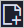This topic describes how to use a for-each node to repeat a loop twice and display the loop count.

Note
• You can only use for-each nodes in DataWorks Standard Edition or higher.
• You can use a for-each node to repeat a loop for a maximum of 128 times. If the loop count exceeds this limit, an error occurs.
• If results need to be traversed based on logical conditions in a for-each node, you can use branch nodes. Note that branch nodes must be used together with merge nodes in a for-each node.

## Create a for-each node

1. Log on to the DataWorks console. In the left-side navigation pane, click Workspaces. On the Workspaces page, find the target workspace and click Data Analytics in the Actions column.
2. Move the pointer over theicon and choose General > for-each.

You can also find the target workflow, right-click General, and choose Create > for-each.

3. In the Create Node dialog box that appears, enter the node name, select the target folder, and click Commit.
Note A node name can be up to 128 characters in length.
4. In the created workflow, create an assignment node as the parent node of the for-each node.
In this example, the assignment node is a Shell node. You can enter the following sample code for the node:
``echo 'this is name,ok';``

The outputs parameter is the default output parameter of the assignment node.

Note
• The start and end nodes of the for-each node have fixed logic and cannot be edited.
• After editing the code of the Shell node, save the modification. No message will appear reminding you to save the modification when you commit the node. If you do not save the message , the latest code cannot be updated in time.
You can enter the following sample code for the Shell node:
``echo \${dag.loopTimes} ----Displays the loop count.``
A for-each node supports the following environment variables:
• \${dag.foreach.current}: the current data row.
• \${dag.loopDataArray}: the input dataset.
• \${dag.offset}: the offset of the loop count to 1.
• \${dag.loopTimes}: the loop count, whose value equals to the value of \${dag.offset} plus 1.
``````// Compare the code of the Shell node with that of a common for loop.
data=[]  // It is equivalent to \${dag.loopDataArray}.
// i is equivalent to \${dag.offset}.
for(int i=0;i<data.length;i++) {
print(data[i]);  // data[i] is equivalent to \${dag.foreach.current}.
}``````

The \${dag.loopDataArray} parameter is the default input parameter of the for-each node. Set this parameter to the value of the outputs parameter of the parent node. If you do not set this parameter, an error occurs when you commit the node.

Commit and deploy the node. Then, go to Operation Center to view the running result.